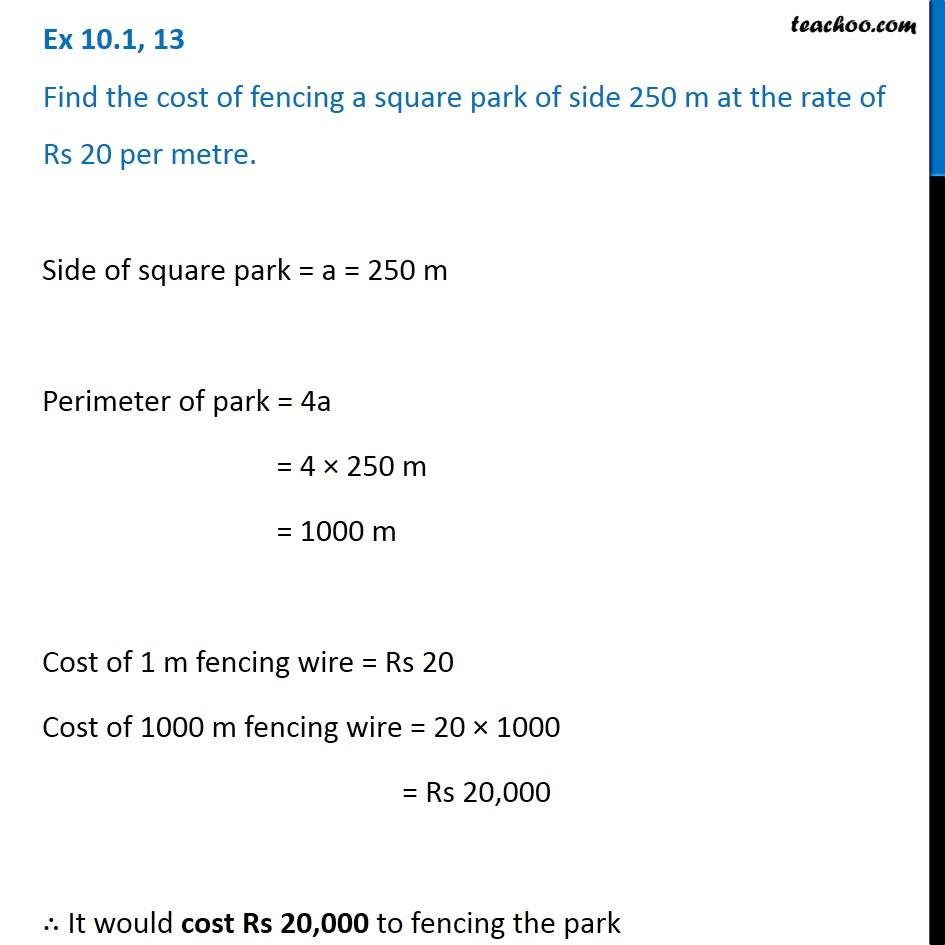Perimeter of Square

Chapter 10 Class 6 Mensuration
Concept wiseLearn in your speed, with individual attention - Teachoo Maths 1-on-1 Class

### Transcript

Ex 10.1, 13 Find the cost of fencing a square park of side 250 m at the rate of Rs 20 per metre. Side of square park = a = 250 m Perimeter of park = 4a = 4 × 250 m = 1000 m Cost of 1 m fencing wire = Rs 20 Cost of 1000 m fencing wire = 20 × 1000 = Rs 20,000 ∴ It would cost Rs 20,000 to fencing the park